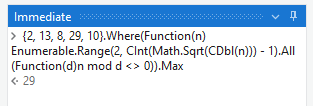# Find largest prime number in a given set of numbers . Can anyone help me please how to do

i have a set of numbers they are 2,13,8,29,10. From these numbers i want to find largest prime number can anyone help me please.

@sandhyareddy

Assign:
largestPrime = 0
numbers = {2, 13, 8, 29, 10}

For Each:
item in numbers

Assign:
isPrime = True

For Each:
num in Enumerable.Range(2, Math.Sqrt(item) - 1)

``````If:
item Mod num = 0
Assign:
isPrime = False
Break (exit the inner For Each loop)
``````

If:
isPrime = True And item > largestPrime
Assign:
largestPrime = item

Write Line:
"largestPrime: " + largestPrime

Try this:
result = numbers.Where(Function(d) IsPrime(d)).Max()

Hey @sandhyareddy
I have uploaded the solution below
Hope it helps you
test.zip (954.1 KB)

1 Like

just hover on For each activity and check if it is string or int

Can you send your sequence here, so that i can check and rectify it
Because the process which i did is working perfectly
So can u please send it here

@sandhyareddy

You can simply use this in an assign activity to get the maximum prime number
result = numbers.Where(Function(d) IsPrime(d)).Max()

IsPrime is a variable or default key

@sandhyareddy

It’s a function. Use invoke code activity to write this code and call this function

``````Dim IsPrime As Func(Of Integer, Boolean) = Function(n)
If n <= 1 Then Return False
If n = 2 Then Return True
If n Mod 2 = 0 Then Return False

Dim sqrtN As Integer = CInt(Math.Sqrt(n))
For i As Integer = 3 To sqrtN Step 2
If n Mod i = 0 Then Return False
Next
Return True
End Function
``````

`IsPrime` is not a default key or variable. Instead, it is likely to be a custom function created by the developer to determine whether a given number is prime.

Also u can use create a IsPrime Variable and give a logic to it

Something like this
for (int i = 2; i < number; i++) {
if (number % i == 0) {
IsPrime = false;
break;
}

First change PrimeNumbers to Int32, then re add for each activity and in list of items enter the variable PrimeNumbers. This will convert it to UiPath.Core.Activities.ForEach<System.Int32>

Could you check the below Expression :

``````{2, 13, 8, 29, 10}.Where(Function(n)Enumerable.Range(2, CInt(Math.Sqrt(CDbl(n))) - 1).All(Function(d)n mod d <> 0)).Max
``````

Visuals :We can substitute the hard coded array with an array variable.

Check out the below workflow:

Sequence15.xaml (8.6 KB)

Hope it helps!!
Regards,

Thankyou! Got it.This topic was automatically closed 3 days after the last reply. New replies are no longer allowed.